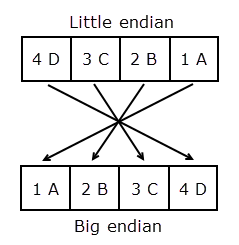Main Content

# swapbytes

Swap byte ordering

## Syntax

``Y = swapbytes(X)``

## Description

example

````Y = swapbytes(X)` swaps the byte ordering of each element in array X from little endian to big endian (or vice versa). All elements of the input array must be full, noncomplex, and numeric.```

## Examples

collapse all

Swap the byte ordering for a scalar 32-bit value. Use hexadecimal representation to show the conversion from little endian to big endian.

```X = uint32(hex2dec('4D3C2B1A')); Y = dec2hex(swapbytes(X))```
```Y = '1A2B3C4D' ```

Starting in R2019b, you can also write a hexadecimal value as a literal using the `0x` or `0X` prefix (for more information, see Hexadecimal and Binary Values). Specify the number `X` as a hexadecimal literal. Swap the byte ordering.

```X = 0x4D3C2B1A; Y = dec2hex(swapbytes(X))```
```Y = '1A2B3C4D' ```

Swap the byte ordering for each element of a 1-by-4 vector.

```format short X = uint16([0 1 128 65535])```
```X = 1x4 uint16 row vector 0 1 128 65535 ```
`Y = swapbytes(X)`
```Y = 1x4 uint16 row vector 0 256 32768 65535 ```

Examine the output in hexadecimal notation to show the endian conversion.

```format hex X```
```X = 1x4 uint16 row vector 0000 0001 0080 ffff ```
`Y`
```Y = 1x4 uint16 row vector 0000 0100 8000 ffff ```

Create a three-dimensional array `A` of 16-bit integers. Swap the byte ordering of each element. Use hexadecimal representation to show the endian conversion.

```format hex X = uint16(magic(3)*150); X(:,:,2) = X*40; X```
```X = 3x3x2 uint16 array X(:,:,1) = 04b0 0096 0384 01c2 02ee 041a 0258 0546 012c X(:,:,2) = bb80 1770 8ca0 4650 7530 a410 5dc0 d2f0 2ee0 ```
`Y = swapbytes(X)`
```Y = 3x3x2 uint16 array Y(:,:,1) = b004 9600 8403 c201 ee02 1a04 5802 4605 2c01 Y(:,:,2) = 80bb 7017 a08c 5046 3075 10a4 c05d f0d2 e02e ```

## Input Arguments

collapse all

Numeric value, specified as a scalar, vector, matrix, or multidimensional array. The `swapbytes` operation is elementwise when `X` is nonscalar.

Data Types: `single` | `double` | `int8` | `int16` | `int32` | `int64` | `uint8` | `uint16` | `uint32` | `uint64`

## More About

collapse all

### Convert Little Endian to Big Endian

Computer systems store data in pieces of bytes. There are two ways to store a numeric value in computer memory: little endian and big endian. In little-endian style, the bytes are written from left to right in increasing significance. In big-endian style, the bytes are written from left to right in decreasing significance. The `swapbytes` function swaps the byte ordering in memory, converting little endian to big endian (and vice versa).

The following figure illustrates the conversion for a 32-bit (4-byte) hexadecimal number, 4D3C2B1A. In hexadecimal notation, one byte is represented by two digits. Each box in the little-endian picture shows one byte of data (4D, 3C, 2B, and 1A). The `swapbytes` function swaps the order of these boxes or bytes.## See Also

Introduced before R2006a

Download ebook